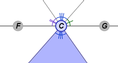# Triangle Angle Sum

### DESCRIPTION

Measure the interior angles of a triangle and find the sum. Examine whether that sum is the same for all triangles. Also, discover how the measure of an exterior angle relates to the interior angle measures.

Full Lesson Info